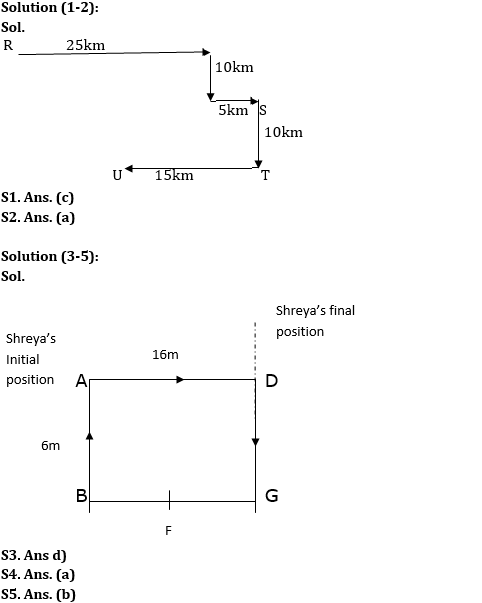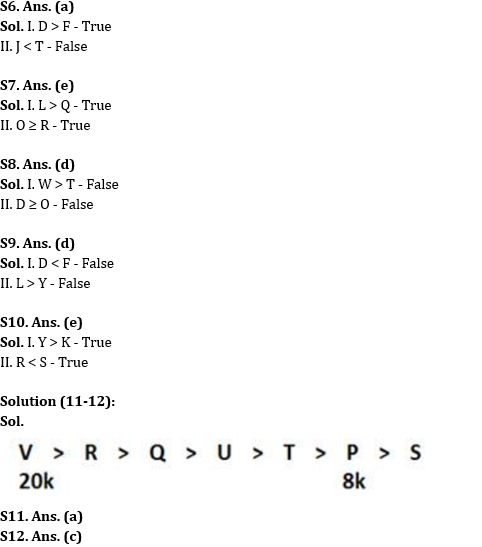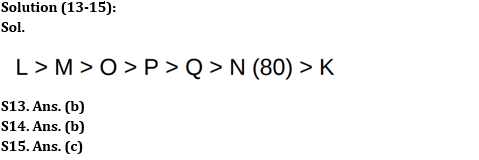Latest Banking jobs   »

# Reasoning Ability Quiz For IDBI 2022- 2nd June

Directions (1-2): Study the information given below and answer the questions based on it:
A person starts his journey from point R. He drives 25 km in east direction and then take a right turn and drives 10km. After taking left turn he reached point S after driving 5 km. Point T is 10 km to the south of S. From T, he is driving 15 km to the west to reach point U. Point S is to the southeast with respect to Point R.

Reasoning Ability Quiz For IDBI 2022 CHECK IN HINDI

Q1. If point K is 20km to the south of R, then what is the shortest distance between K and U?
(a) 20km
(b) 25km
(c) 15km
(d) 30km
(e) 35km

Q2. What is the direction of point U with respect to point S?
(a) South-west
(b) South-east
(c) East
(d) North-west
(e) None of these

Directions (3-5): Study the information given below and answer the questions based on it:
Point A is 6m to the north of point B. Point D is 16m to the east of point A. Point F is exactly in the middle of B and G. G is 6m south of D. Shreya is standing at point A, she walks 16m towards the east and then turns to her left and walks 6m.

Q3. In which direction is Shreya now with respect to her initial position?
(a) South-west
(b) South-east
(c) East
(d) North-east
(e) None of these

Q4. What is the shortest distance between D and F?
(a) 10m
(b) 15m
(c) 20m
(d) 30m
(e) 35m

Q5. What is the direction of point G with respect to point A?
(a) South-west
(b) South-east
(c) East
(d) North-west
(e) None of these

Directions (6-10): In the following questions assuming the given statement to be true, find which of the conclusion(s) among given conclusions is/are definitely true and then give your answers accordingly.

Q6. Statement: D > L ≥ J ≥ F ≤ U < G ≤ T
Conclusions:
I. D > F
II. J < T
(a) If only conclusion I is true
(b) If only conclusion II is true
(c) If either conclusion I or II is true
(d) If neither conclusion I nor II is true
(e) If both conclusions I and II are true

Q7. Statement: L ≥ C > D ≥ E = R; O ≥ D ≥ Q
Conclusions:
I. L > Q
II. O ≥ R
(a) If only conclusion I is true
(b) If only conclusion II is true
(c) If either conclusion I or II is true
(d) If neither conclusion I nor II is true
(e) If both conclusions I and II are true

Q8. Statements: N < D ≥ W; O ≥ T > G; N > O
Conclusions:
I. W > T
II. D ≥ O
(a) If only conclusion I is true
(b) If only conclusion II is true
(c) If either conclusion I or II is true
(d) If neither conclusion I nor II is true
(e) If both conclusions I and II are true

Q9. Statement: Y < O < D > I = L > F
Conclusions:
I. D < F
II. L > Y
(a) If only conclusion I is true
(b) If only conclusion II is true
(c) If either conclusion I or II is true
(d) If neither conclusion I nor II is true
(e) If both conclusions I and II are true

Q10. Statements: A < K < D > R; Y > D < S
Conclusions:
I. Y > K
II. R < S
(a) If only conclusion I is true
(b) If only conclusion II is true
(c) If either conclusion I or II is true
(d) If neither conclusion I nor II is true
(e) If both conclusions I and II are true

Directions (11-12): Study the following information carefully and answer the given questions.
Seven persons i.e., P, Q, R, S, T, U and V earns different salaries. Q earns more than T but less than only two persons. P earns more than S but less than T. R earns more than Q but does not earn highest. U earns more than T but less than Q. The one who earns highest salary, earns 20k and the one who earns second lowest salary, earns 8k.

Q11. Who amongst the following earns second highest salary?
(a) R
(b) V
(c) U
(d) T
(e) None of these

Q12. What may be the possible salary of S?
(a) 13k
(b) 10k
(c) 7k
(d) 12k
(e) None of these

Directions (13-15): Study the following information carefully and answer the given questions.
There are seven people i.e., K, L, M, N, O, P and Q having different height. The height of second shortest person is 80 cm. There are two persons between M and Q who is shorter than M. P is taller than K but shorter than O. L is taller than N. N is shorter than Q but not the shortest person. M is taller than O but not the tallest person.

Q13. Who amongst the following is the second tallest person?
(a) O
(b) M
(c) L
(d) P
(e) None of these

Q14. How many people are taller than N?
(a) Four
(b) Five
(c) Three
(d) Two
(e) None of these

Q15. If the height of P is 92 cm, then what will be the possible height of Q?
(a) 93 cm
(b) 79 cm
(c) 87 cm
(d) 78 cm
(e) None of these

Solutions#### Congratulations!